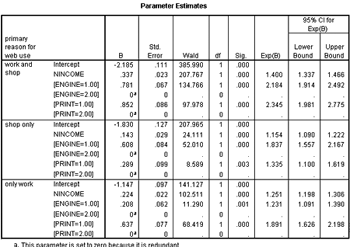# IBM SPSS Regression

### Improve Predictions with Powerful Nonlinear Regression Software

• Overview
• Features and Benefits

IBM® SPSS® Regression enables you to predict categorical outcomes and apply a wide range of nonlinear regression procedures.

You can apply IBM SPSS Regression to many business and analysis projects where ordinary regression techniques are limiting or inappropriate: for example, studying consumer buying habits or responses to treatments, measuring academic achievement, and analyzing credit risks.

IBM SPSS Regression includes the following procedures:

• Multinomial logistic regression: Predict categorical outcomes with more than two categories
• Binary logistic regression: Easily classify your data into two groups
• Nonlinear regression and constrained nonlinear regression (CNLR): Estimate parameters of nonlinear models
• Weighted least squares: Gives more weight to measurements within a series
• Two-stage least squares: Helps control for correlations between predictor variables and error terms
• Probit analysis: Evaluate the value of stimuli using a logit or probit transformation of the proportion responding

### More Statistics for Data Analysis

Expand the capabilities of IBM® SPSS® Statistics Base for the data analysis stage in the analytical process. Using IBM SPSS Regression with IBM SPSS Statistics Base gives you an even wider range of statistics so you can get the most accurate response for specific data types.

IBM SPSS Regression includes:

• Multinomial logistic regression (MLR): Regress a categorical dependent variable with more than two categories on a set of independent variables. This procedure helps you accurately predict group membership within key groups.
You can also use stepwise functionality, including forward entry, backward elimination, forward stepwise or backward stepwise, to find the best predictor from dozens of possible predictors. If you have a large number of predictors, Score and Wald methods can help you more quickly reach results. You can access your model fit using Akaike information criterion (AIC) and Bayesian information criterion (BIC; also called Schwarz Bayesian criterion, or SBC).
• Binary logistic regression: Group people with respect to their predicted action. Use this procedure if you need to build models in which the dependent variable is dichotomous (for example, buy versus not buy, pay versus default, graduate versus not graduate). You can also use binary logistic regression to predict the probability of events such as solicitation responses or program participation.
With binary logistic regression, you can select variables using six types of stepwise methods, including forward (the procedure selects the strongest variables until there are no more significant predictors in the dataset) and backward (at each step, the procedure removes the least significant predictor in the dataset) methods. You can also set inclusion or exclusion criteria. The procedure produces a report telling you the action it took at each step to determine your variables.
• Nonlinear regression (NLR) and constrained nonlinear regression (CNLR): Estimate nonlinear equations. If you are you working with models that have nonlinear relationships, for example, if you are predicting coupon redemption as a function of time and number of coupons distributed, estimate nonlinear equations using one of two IBM SPSS Statistics procedures: nonlinear regression (NLR) for unconstrained problems and constrained nonlinear regression (CNLR) for both constrained and unconstrained problems.
NLR enables you to estimate models with arbitrary relationships between independent and dependent variables using iterative estimation algorithms, while CNLR enables you to:
• Use linear and nonlinear constraints on any combination of parameters
• Estimate parameters by minimizing any smooth loss function (objective function)
• Compute bootstrap estimates of parameter standard errors and correlations
• Weighted least squares (WLS): If the spread of residuals is not constant, the estimated standard errors will not be valid. Use Weighted Least Square to estimate the model instead (for example, when predicting stock values, stocks with higher shares values fluctuate more than low value shares.)
• Two-stage least squares (2LS): Use this technique to estimate your dependent variable when the independent variables are correlated with the regression error terms.
For example, a book club may want to model the amount they cross-sell to members using the amount that members spend on books as a predictor. However, money spent on other items is money not spent on books, so an increase in cross-sales corresponds to a decrease in book sales. Two-Stage Least-Squares Regression corrects for this error.
• Probit analysis: Probit analysis is most appropriate when you want to estimate the effects of one or more independent variables on a categorical dependent variable.
For example, you would use probit analysis to establish the relationship between the percentage taken off a product, and whether a customer will buy as the prices decreases. Then, for every percent taken off the price you can work out the probability that a consumer will buy the product.
• IBM SPSS Regression includes additional diagnostics for use when developing a classification table.The multinomial logistic regression procedure predicts a categorical outcome such as "primary reason for Web use." The categories in this example are: a) work only, b) shopping only, c) both working and shopping, and d) neither (reference category). From the results above, we can see that search engine use was a better predictor of "shopping only" than print media use.

The multinomial logistic regression procedure predicts a categorical outcome such as "primary reason for Web use." The categories in this example are: a) work only, b) shopping only, c) both working and shopping, and d) neither (reference category). From the results above, we can see that search engine use was a better predictor of "shopping only" than print media use.